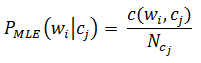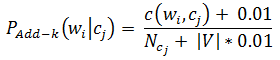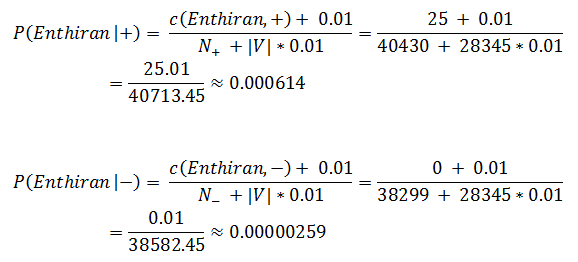Please visit, subscribe and share 10 Minutes Lectures in Computer Science

## Naive Bayes Classifier Solved Exercise

Question:
Assume that a Naive Bayes classifier has a vocabulary that consists of 28345 word types. Suppose that training the classifier on a collection of movie reviews gave us the following;
count(Enthiran, +) = 25, count(Enthiran, −) = 0, 𝑁+ =40430, 𝑁 = 38299
Here, count(𝑤,𝑐) gives us the count of occurrences of 𝑤 in documents that are under class 𝑐, + refers to positive reviews class, refers to negative reviews class and 𝑁𝑐 refers to the total number of word occurrences in documents with class 𝑐. Estimate 𝑃(Enthiran | +) and 𝑃(Enthiran | −) using Maximum Likelihood estimation with Add-k smoothing, with 𝑘=0.01.

Solution:
Given,
|V| = 28345
count(Enthiran, +) = 25
count(Enthiran, −) = 0
𝑁+ =40430
𝑁 = 38299.
As per maximum likelihood estimate, the bi-gram probability can be calculated as follows;Also, it is said that we need to Add-k smoothing with k = 0.01. Hence, the above equation can be modified to smooth as follows;With this equation, we can calculate the probabilities;*********

Go to NLP Glossary